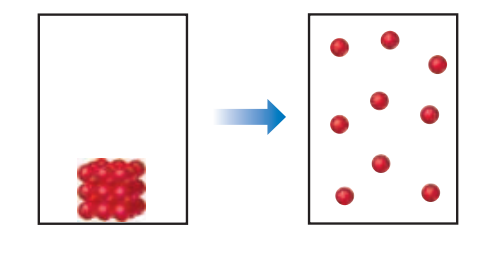×
Get Full Access to Chemistry: The Central Science - 14 Edition - Chapter 19 - Problem 19.30
Get Full Access to Chemistry: The Central Science - 14 Edition - Chapter 19 - Problem 19.30

×ISBN: 9780134414232 1274

## Solution for problem 19.30 Chapter 19

Chemistry: The Central Science | 14th Edition

• Textbook Solutions
• 2901 Step-by-step solutions solved by professors and subject experts
• Get 24/7 help from StudySoup virtual teaching assistantsChemistry: The Central Science | 14th Edition

4 5 1 414 Reviews
28
2
Problem 19.30

(a) What are the signs of $$\Delta S$$ and $$\Delta H$$ for the process depicted here?

(b) If energy can flow in and out of the system to maintain a constant temperature during the process, what can you say about the entropy change of the surroundings as a result of this process? [Sections 19.2 and 19.5]Text Transcription:

\Delta S

\Delta H

Step-by-Step Solution:
Step 1 of 3

Antavia Tinker BIO 101 Royster 9.18.2018 Introduction to Acids & Bases: A Web Quest 1. http://www.visionlearning.com/library/module_viewer.phpc3=&mid=58&l= The word acid comes from the Latin word acere meaning sour. Boyle stated that acids taste sour, are corrosive to metals, change the color of litmus to red, and become less acidic when mixed with bases. He described bases as feeling slippery, changing litmus to the color blue, and becoming less basic when mixed with an acid. About 200 years later, Arrhenius proposed that water can dissolve many compounds by separating them into their individual ions. He suggested that acids contain hydrogen and can dissolve in water to release hydrogen ions. Bases dissolve in water to release hydroxide ions into the solution. 2. http://www.chem4kids.com/files/react_acidbase.html Every liquid has acidic& basic traits. One exception might be H2O. It is just water. However, the positive ions and negative ions cancel each other out. 3. http://chemistry.about.com/od/acidsbases/a/acidbaseformula.htm Give the formula for the following acids: Hydrofluoric Acid­HF Hydrochloric Acid­HCl Hydro sulfuric Acid­H S 2 Nitric Acid­HNO 3 Sulfuric Acid­H2 OS 4 Acetic Acid­HC H 2 3 2 Boric Acid­H B3 3 Give the formula for the following bases: Sodium Hydroxide­NaOH Potassium Hydroxide­KOH Calcium Hydroxide­Ca(OH )2 Iron (II) Hydroxide­Fe(OH

Step 2 of 3

Step 3 of 3

## Discover and learn what students are asking

Calculus: Early Transcendental Functions : Area and Arc Length in Polar Coordinates
?In Exercises 5-16, find the area of the region. Interior of $$r=3 \cos \theta$$

Statistics: Informed Decisions Using Data : Applications of the Normal Distribution
?Give three interpretations for the area under a normal curve.

Chemistry: The Central Science : Chemical Reactions and Reaction Stoichiometry
?The compound $$\mathrm{XCl}_{4}$$ contains 75.0 % CI by mass. What is the element X?

Unlock Textbook Solution